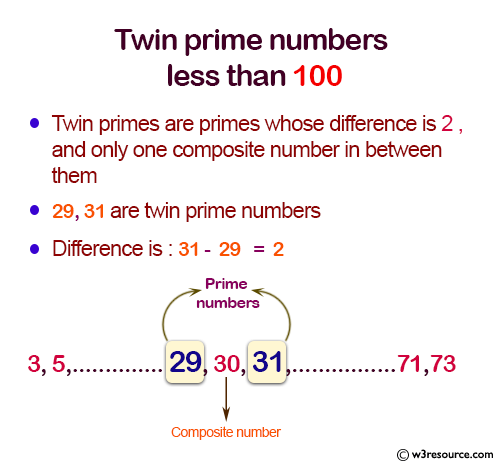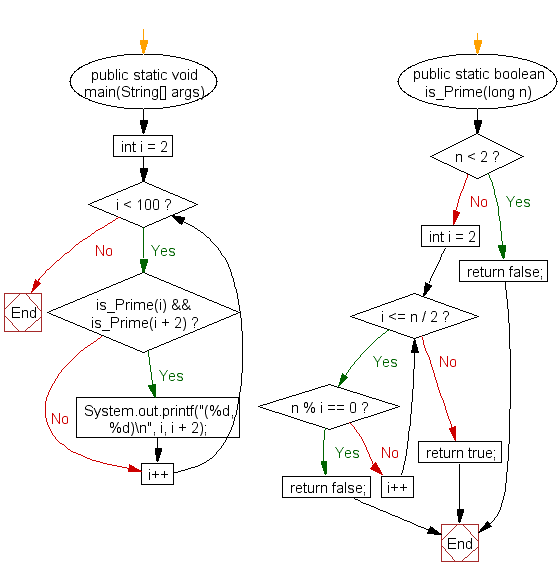﻿ Java exercises: Find all twin prime numbers less than 100 - w3resource# Java Method Exercises: Find all twin prime numbers less than 100

## Java Method: Exercise-16 with Solution

Write a Java method to find all twin prime numbers less than 100.

Pictorial Presentation:Sample Solution:

Java Code:

``````import java.util.Scanner;
public class Exercise16 {

public static void main(String[] args) {

for (int i = 2; i < 100; i++) {

if (is_Prime(i) && is_Prime(i + 2)) {
System.out.printf("(%d, %d)\n", i, i + 2);
}
}
}

public static boolean is_Prime(long n) {

if (n < 2) return false;

for (int i = 2; i <= n / 2; i++) {

if (n % i == 0) return false;
}
return true;
}

}
```
```

Sample Output:

```(3, 5)
(5, 7)
(11, 13)
(17, 19)
(29, 31)
(41, 43)
(59, 61)
(71, 73)
```

Flowchart :Java Code Editor: